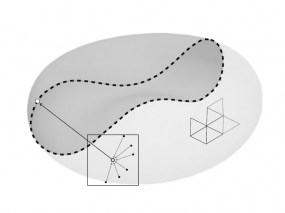# Lorena A. Barba groupLoading...

## Submitted: Inexact Krylov iterations and relaxation strategies with fast-multipole boundary element methodSubmitted: 19 June 2015   This paper presents a method to algorithmically accelerate boundary element methods. The fast multipole method (FMM) is already used in this context to obtain O(N) computational complexity while solving a dense linear system. Using relaxed-accuracy matrix-vector multiplications in a Krylov solver, we can achieve speed-ups in the order of 4x... Continue »

## Validation of the PyGBe code for Poisson-Boltzmann equation

The PyGBe code solves the linearized Poisson-Boltzmann equation using a boundary-integral formulation. We use a boundary element method with a collocation approach, and solve it via a Krylov-subspace method. To do this efficiently, the matrix-vector multiplications in the Krylov iterations are accelerated with a... Continue »